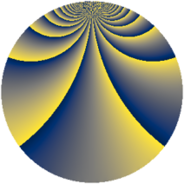# Properties

 Label 2268.1.sLevel $2268$ Weight $1$ Character orbit 2268.s Rep. character $\chi_{2268}(755,\cdot)$ Character field $\Q(\zeta_{6})$ Dimension $32$ Newform subspaces $7$ Sturm bound $432$ Trace bound $28$

# Learn more

## Defining parameters

 Level: $$N$$ $$=$$ $$2268 = 2^{2} \cdot 3^{4} \cdot 7$$ Weight: $$k$$ $$=$$ $$1$$ Character orbit: $$[\chi]$$ $$=$$ 2268.s (of order $$6$$ and degree $$2$$) Character conductor: $$\operatorname{cond}(\chi)$$ $$=$$ $$252$$ Character field: $$\Q(\zeta_{6})$$ Newform subspaces: $$7$$ Sturm bound: $$432$$ Trace bound: $$28$$

## Dimensions

The following table gives the dimensions of various subspaces of $$M_{1}(2268, [\chi])$$.

Total New Old
Modular forms 80 40 40
Cusp forms 32 32 0
Eisenstein series 48 8 40

The following table gives the dimensions of subspaces with specified projective image type.

$$D_n$$ $$A_4$$ $$S_4$$ $$A_5$$
Dimension 32 0 0 0

## Trace form

 $$32q - 4q^{4} + O(q^{10})$$ $$32q - 4q^{4} - 4q^{16} + 4q^{22} + 12q^{25} - 8q^{37} - 8q^{46} + 8q^{49} + 8q^{64} + 4q^{70} + 8q^{85} + 4q^{88} + O(q^{100})$$

## Decomposition of $$S_{1}^{\mathrm{new}}(2268, [\chi])$$ into newform subspaces

Label Dim. $$A$$ Field Image CM RM Traces $q$-expansion
$$a_2$$ $$a_3$$ $$a_5$$ $$a_7$$
2268.1.s.a $$2$$ $$1.132$$ $$\Q(\sqrt{-3})$$ $$D_{3}$$ $$\Q(\sqrt{-21})$$ None $$-1$$ $$0$$ $$-1$$ $$1$$ $$q-\zeta_{6}q^{2}+\zeta_{6}^{2}q^{4}+\zeta_{6}^{2}q^{5}+\zeta_{6}q^{7}+\cdots$$
2268.1.s.b $$2$$ $$1.132$$ $$\Q(\sqrt{-3})$$ $$D_{3}$$ $$\Q(\sqrt{-21})$$ None $$-1$$ $$0$$ $$1$$ $$-1$$ $$q-\zeta_{6}q^{2}+\zeta_{6}^{2}q^{4}-\zeta_{6}^{2}q^{5}-\zeta_{6}q^{7}+\cdots$$
2268.1.s.c $$2$$ $$1.132$$ $$\Q(\sqrt{-3})$$ $$D_{3}$$ $$\Q(\sqrt{-21})$$ None $$1$$ $$0$$ $$-1$$ $$-1$$ $$q+\zeta_{6}q^{2}+\zeta_{6}^{2}q^{4}+\zeta_{6}^{2}q^{5}-\zeta_{6}q^{7}+\cdots$$
2268.1.s.d $$2$$ $$1.132$$ $$\Q(\sqrt{-3})$$ $$D_{3}$$ $$\Q(\sqrt{-21})$$ None $$1$$ $$0$$ $$1$$ $$1$$ $$q+\zeta_{6}q^{2}+\zeta_{6}^{2}q^{4}-\zeta_{6}^{2}q^{5}+\zeta_{6}q^{7}+\cdots$$
2268.1.s.e $$8$$ $$1.132$$ $$\Q(\zeta_{24})$$ $$D_{12}$$ $$\Q(\sqrt{-7})$$ None $$0$$ $$0$$ $$0$$ $$0$$ $$q+\zeta_{24}^{9}q^{2}-\zeta_{24}^{6}q^{4}-\zeta_{24}^{2}q^{7}+\cdots$$
2268.1.s.f $$8$$ $$1.132$$ $$\Q(\zeta_{24})$$ $$D_{12}$$ $$\Q(\sqrt{-7})$$ None $$0$$ $$0$$ $$0$$ $$0$$ $$q-\zeta_{24}^{7}q^{2}-\zeta_{24}^{2}q^{4}+\zeta_{24}^{2}q^{7}+\cdots$$
2268.1.s.g $$8$$ $$1.132$$ $$\Q(\zeta_{24})$$ $$D_{4}$$ $$\Q(\sqrt{-7})$$ None $$0$$ $$0$$ $$0$$ $$0$$ $$q-\zeta_{24}^{5}q^{2}+\zeta_{24}^{10}q^{4}-\zeta_{24}^{2}q^{7}+\cdots$$